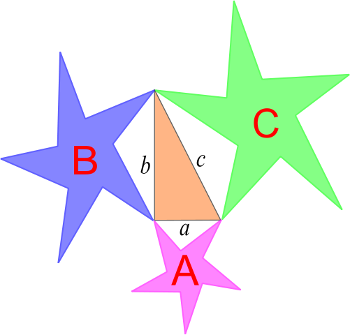# Equal OpportunityThe Pythagorean theorem works for any similar shapes, not just squares.

In the figure above, A + B = C.

If the three sides of a right triangle are made the diameters of three circles, then the combined area of the two smaller circles equals that of the largest. That’s also the area of the circumcircle, since a right triangle’s hypotenuse forms the diameter of its circumscribing circle.